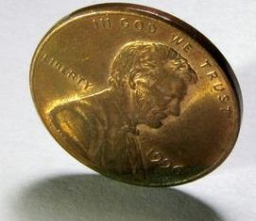# A fair coin

A fair coin is tossed twice. Write down the set of possible outcomes.
What is the probability of obtaining?

p1 =  0.5
p2 =  0.25

### Step-by-step explanation:Did you find an error or inaccuracy? Feel free to write us. Thank you!

Tips for related online calculators
Need help calculating sum, simplifying, or multiplying fractions? Try our fraction calculator.